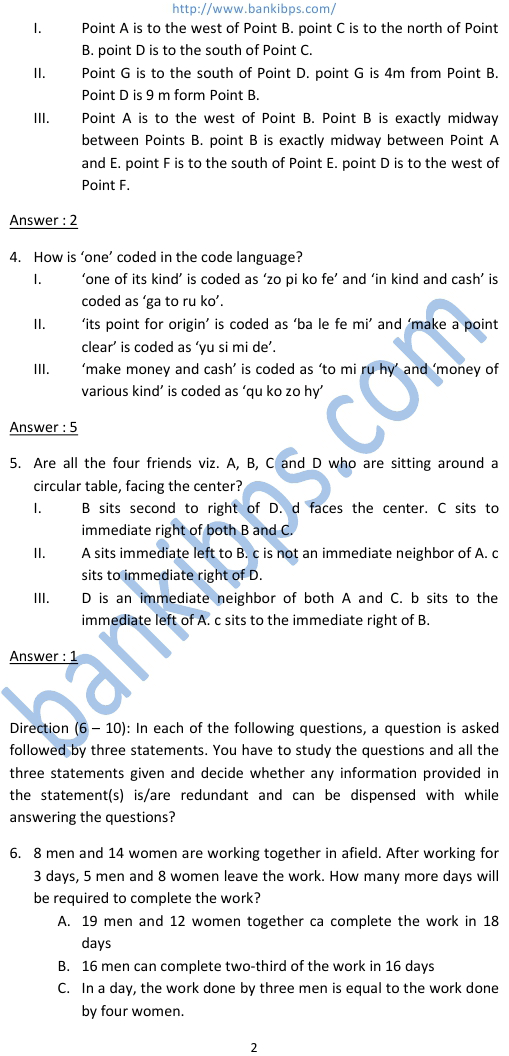# Data Sufficiency Practice

Direction (1 – 5): Each of the questions below consists of a question and three statements numbered I, II and III given below it. You have to decide whether the data provided in the statements are sufficient to answer the question. Read all the three statements and – data sufficiency practice Give answer (1) if the data in statement I and II are sufficient to answer the question, while the data in Statement III are not required to answer the questions. Give answer (2) if the data in Statement I and III are sufficient to answer the question, while the data in Statement II are not required to answer the question. Give answer (3) if the data in Statement II and III are sufficient to answer the question, while the data in Statement I are not required to answer the question. Give answer (4) if the data in either Statement I alone or Statement II alone or Statement III alone are sufficient to answer the question. Give answer (5) if the data in all the Statement I, II and III together are necessary to answer the question. 1. Among six people P, Q, R, S, T and V each lives on a different floor of a six storey building having six floor numbered one to six (the ground floor is numbered 1, the floor above it numbered 2 and so on and the topmost floor is numbered 6). Who lives on the topmost floor? I. There is only one floor between the floors on which R and Q lives on an even numbered floor. II. T does not live on an even numbered floor. Q lives on an even numbered floor. Q does not live on the topmost floor. III. S lives on an odd numbered floor. There are two floor between the floor on which S and P live. T lives on a floor immediately above R’s floor. Answer : 5 2. There are six letters W, A, R, S, N and E. is ‘ANSWER’ the word formed after performing the following operations using these six letters only? I. E is placed fourth to the right of A. S is not placed immediately next to either A or E. II. R is placed immediately next (either left or right) to E. W is placed immediately next (either left or right) to S. III. Both N and W are placed immediately next to S. the word does not begin with R. A is not placed immediately next to W.
Practice Exercise - 329 [ Data Sufficiency ]## data sufficiency practice

### data sufficiency practice test

#### data sufficiency tutorial

##### data sufficiency preparation
###### gmat data sufficiency tests
data sufficiency practice test. data sufficiency tutorial. data sufficiency preparation. gmat data sufficiency tests. cat data sufficiency sample test data sufficiency practice questions. data sufficiency gmat. sample gmat data sufficiency questions. data sufficiency practice questions pdf. data sufficiency practice problems. free data sufficiency practice questions.